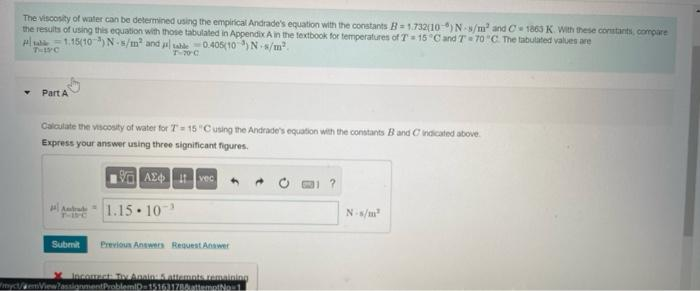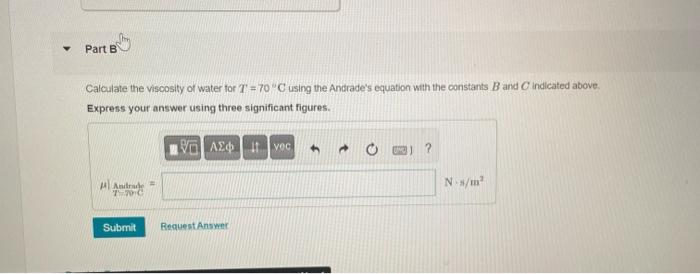Home / Expert Answers / Civil Engineering / pleasw-help-witht-the-following-question-thank-you-for-your-time-nbsp-the-results-of-using-this-e-pa754

# (Solved): pleasw help witht the following question. thank you for your time.  the results of using this e ...

pleasw help witht the following question. thank you for your time.the results of using this equation with those tabulated in AppendixA in the fextbock for temperatures of $$T=15^{\circ} \mathrm{C}$$ and $$T$$ = $$70^{\circ} \mathrm{C}$$. The tabulated values are - Part A Express your answer using three significant figures. Calculate the viscosity of water for $$T=70^{*} \mathrm{C}$$ using the Andrade's equation with the constants $$B$$ and $$C$$ indicated above. Express your answer using three significant figures.

We have an Answer from Expert